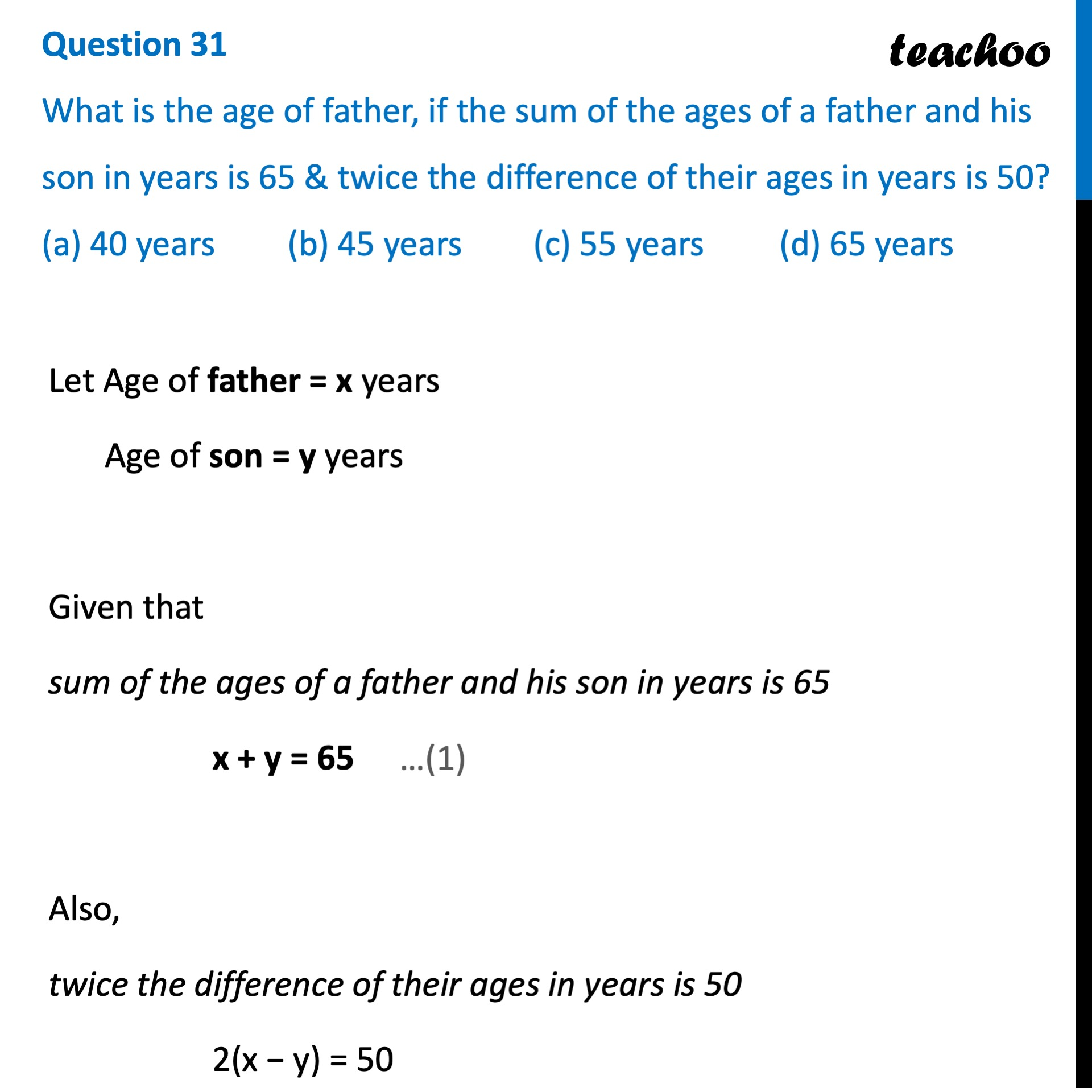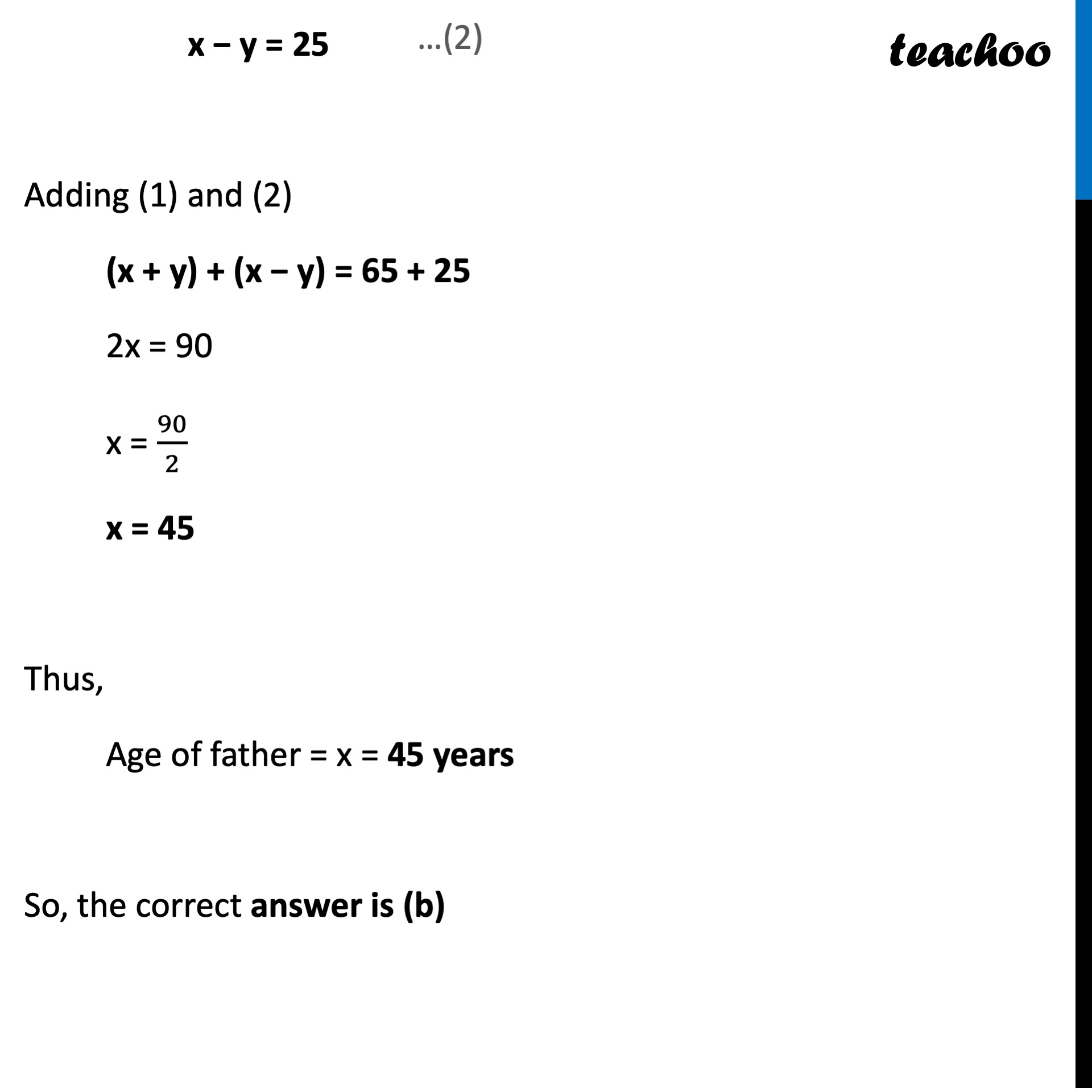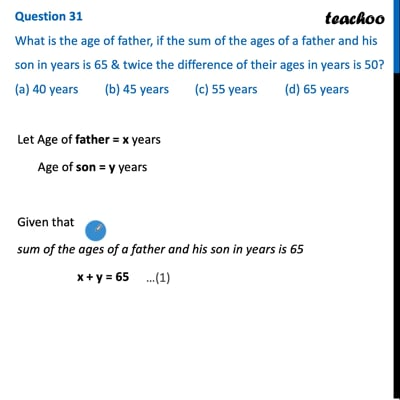CBSE Class 10 Sample Paper for 2022 Boards - Maths Basic [MCQ]

Class 10
Solutions of Sample Papers for Class 10 Boards

## (a) 40 years   (b) 45 years   (c) 55 years   (d) 65 yearsThis video is only available for Teachoo black users

Learn in your speed, with individual attention - Teachoo Maths 1-on-1 Class

### Transcript

Question 31 What is the age of father, if the sum of the ages of a father and his son in years is 65 & twice the difference of their ages in years is 50? (a) 40 years (b) 45 years (c) 55 years (d) 65 years Let Age of father = x years Age of son = y years Given that sum of the ages of a father and his son in years is 65 x + y = 65 Also, twice the difference of their ages in years is 50 2(x − y) = 50 x − y = 25 Adding (1) and (2) (x + y) + (x − y) = 65 + 25 2x = 90 x = 90/2 x = 45 Thus, Age of father = x = 45 years So, the correct answer is (b)